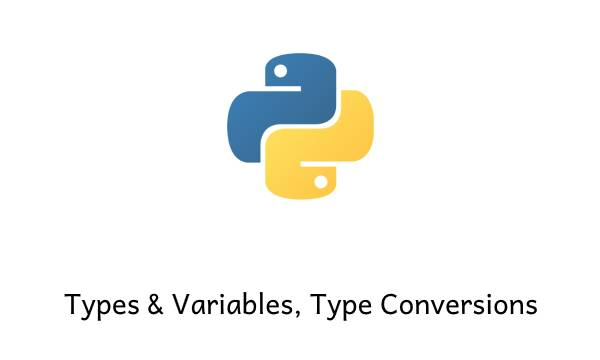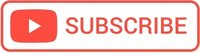# Python Variables & types, Operators, Type Conversion## Variables and Assignment Operators



### Python Identity Operators

In Python Identity operators are

is – evaluates if both sides have the same identity. Is operator is stronger than ==.

is not – evaluates if both sides have different identities

!Comparisons to singletons like None should always be done with ‘is’ or ‘is not’, never with the equality operators. Read more here – Style Guide for Python Code

someobj = None
if not someobj:
#do something

#### Examples

a = [1, 1, 2]
b = a

print(a == b)
# True

print(a is b)
# True


List a and list b are equal and identical.

a = [1, 1, 2]
b = [1, 1, 2]

print(a == b)
# True They have the same content!

print(a is b)
# False


They have the same content but point to two different objects, so they aren’t identical objects.

a = [1, 1, 2]
b = [1, 1, 2, 4]

print(a == b)
# False They don't have the same content!

print(a is b)
# False They don't have the same content and point to different objects!


#### In PHP identical operator is ===

In PHP the Identical operator is the triple equal sign “===”

This operator returns true if both variable contains same information and same data types otherwise return false.

##### Why is === faster than == in PHP?

=== is faster because don’t converts the data type to see if two variables have same value

### Python Bitwise Operators

& AND (Sets each bit to 1 if both bits are 1)
| OR (Sets each bit to 1 if one of two bits is 1)
^ XOR (Sets each bit to 1 if only one of two bits is 1)
~ NOT (Inverts all the bits)
<< Zero fill left shift (Shift left by pushing zeros in from the right and let the leftmost bits fall off)
>> Signed right shift (Shift right by pushing copies of the leftmost bit in from the left, and let the rightmost bits fall off)

### Divide By Zero in Python

What happens if you divide by zero in Python?

ZeroDivisionError: division by zero

## Python Booleans

You can evaluate any expression in Python, and get one of two answers, True or False.

### bool() function

The bool() function allows you to evaluate any value, and give you True or False in return

print(bool("abc"))
# True
print(bool(10))
# True


## None Keyword

The None keyword is used to define a null value or no value at all.
None is a variable, a value, that we can distinctly detect differently than numbers.

None is not the same as 0, False, or an empty string. None is a datatype of its own (NoneType) and only None can be None.

x = None
print(x)

//None


## How do we get data from the user in Python?

You can read data from the user using the input() function.
The input() function returns a string!

name = input(“What is Your name?”)

Even if the user will tipe “123” the result will be string, not int!

## Strings

Strings in Python are arrays of bytes representing Unicode characters.

In Python, You can define a string with either double quotes ” or single quotes ‘.

In Python, a string is immutable. You cannot overwrite the values of immutable objects.

Read more here about Working with strings in Python ✅ Methods, Slicing, String Mutations ✅ Exercises, and examples

## Type & Type Conversion

int + float = float

print(.1 + .1 + .1 + .1 == .4)
False

Because the float, 0.1 is actually slightly more than 0.1.
When we add several of them together will see the difference between the mathematically correct answer and the one that Python creates.

print(0 + 7)
# 7
print("0" + "7")
# 07
print("0" + 7)
# TypeError: can only concatenate str (not "int") to str
print(0 + "7")
TypeError: unsupported operand type(s) for +: 'int' and 'str'


### type() function

The type() function returns the type of the specified object

print(type(10))
# <class 'int'>
print(type("10"))
# <class 'str'>
print(type(10.))
# <class 'float'>


### Type Conversion

You can convert from one type to another with the int(), float(), str() and complex() methods:

#convert from float to int:
a = int(2.0)
print(type(a))
# <class 'int'>

#convert from string to int:
a = str(10)
print(type(a))
# <class 'str'>


#### Hello there!

I hope you find this post useful!

I'm Mihai, a programmer and online marketing specialist, very passionate about everything that means online marketing, focused on eCommerce.

If you have a collaboration proposal or need helps with your projects feel free to contact me. I will always be glad to help you!